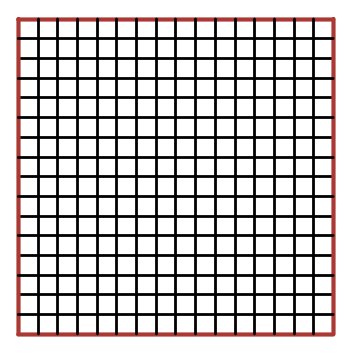# Red Perimeter 5 – Big Square

Probability Level 2Given that an $n \times n$ square made of unit squares has been painted red on its perimeter, and that there are at least 1000 unit squares with no red sides, what is the smallest value $n$ could be?

This problem is part of Arron's set The Red Perimeter.

×